Courses

# Chemistry Test 8 - Mole Concept, Atomic Structure, Periodic Properties, Nomenclature, Isomerism

## 45 Questions MCQ Test NEET Mock Test Series & Past Year Papers | Chemistry Test 8 - Mole Concept, Atomic Structure, Periodic Properties, Nomenclature, Isomerism

Description
This mock test of Chemistry Test 8 - Mole Concept, Atomic Structure, Periodic Properties, Nomenclature, Isomerism for NEET helps you for every NEET entrance exam. This contains 45 Multiple Choice Questions for NEET Chemistry Test 8 - Mole Concept, Atomic Structure, Periodic Properties, Nomenclature, Isomerism (mcq) to study with solutions a complete question bank. The solved questions answers in this Chemistry Test 8 - Mole Concept, Atomic Structure, Periodic Properties, Nomenclature, Isomerism quiz give you a good mix of easy questions and tough questions. NEET students definitely take this Chemistry Test 8 - Mole Concept, Atomic Structure, Periodic Properties, Nomenclature, Isomerism exercise for a better result in the exam. You can find other Chemistry Test 8 - Mole Concept, Atomic Structure, Periodic Properties, Nomenclature, Isomerism extra questions, long questions & short questions for NEET on EduRev as well by searching above.
QUESTION: 1

Solution:
QUESTION: 2

Solution:
QUESTION: 3

### For a neutral unexcited atom, consider four electrons identified by the quantum number n and l :(i) n = 4, l = 1 (ii) n = 4, l = 0(iii) n = 3, l = 2 (iv) n = 3, l = 1 They can be placed in order of increasing energy as

Solution:
QUESTION: 4
Which of the following pairs will combine through a coordinate bond?
Solution:
QUESTION: 5
The total number of covalent bonds present in the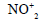ion is
Solution:
QUESTION: 6
The number of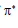electrons in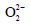is
Solution:
QUESTION: 7
A square planar complex is formed by the hybridization of
Solution:
QUESTION: 8
The density of a gas will be the highest at
Solution:
QUESTION: 9

The hydrated salt Na2CO3 x H2O undergoes 63 % loss in mass on heating and become anhydrous. The value of x is:

Solution:
QUESTION: 10
If the enthalpy of vaporization of water is 186.5 J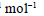the entropy of its vaporization will be
Solution:
QUESTION: 11
The entropy change for a phase transformation is
Solution:
QUESTION: 12
The standard molar enthalpies of formation of ethane(g),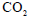(g),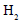O(I) are –21.1 kacl, –94.1
kcal and –68.3 kcal respectively. The standard molar enthalpy of combustion of ethane will be
Solution:
QUESTION: 13
If at 298 K the bond energies of C–H, C–C, C=C and H–H bonds are 414 kJ,347 kJ,
615 kJand 435 kJrespectively, the value of enthalpy change for the reaction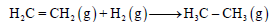at 298 K will be
Solution:
QUESTION: 14

In which of the following reactions is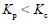?

Solution:
QUESTION: 15

When 1 mole of ethanol is mixed with 1 mole of acetic acid, 0.67 mole of ethyl acetate is produced at equilibrium. Calculate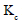for the reaction?

Solution:
QUESTION: 16
When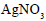is heated in a closed vessel,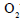gas is liberated and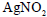is left behind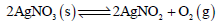At equilibrium
Solution:
QUESTION: 17

The degree of dissociation of 0.1 M  CH3CO2H is (given  Ka ≈ 1.0 x 10-5)

Solution:
QUESTION: 18
Which of the following is the correct representation of the solubility product constant for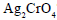Solution:
QUESTION: 19
When equal volumes of the following solutions are mixed, the precipitation of AgCl will occur only with (given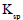of AgCl =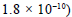Solution:
QUESTION: 20
For a weak monoprotic acid, the pH of a given concentration is calculated by the equation
Solution:
QUESTION: 21
Hydrogen has the tendency to gain one electron to acquire helium configuration, in this respect it
resembles
Solution:
QUESTION: 22
Which of the following is not a water softener?
Solution:
QUESTION: 23
A commercial sample of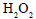can be slowed down by the addition of small amount of phosphoric acid which acts as
Solution:
QUESTION: 24
The IUPAC name of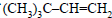is
Solution:
QUESTION: 25

The IUPAC name of  CH3-CH(OH)-CH2-CH(CH)3-CH2-CH3 is

Solution:
QUESTION: 26
The total number of isomers having the molecular formula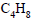is
Solution:
QUESTION: 27
The compound which is not isomeric with diethyl ether is
Solution:
QUESTION: 28
Which of the following exhibits tautomerism?
Solution:
QUESTION: 29
Which of the following compounds will not show cistrans isomerism?
Solution:
QUESTION: 30
Homolytic fission of carbon-carbon bond of ethane produces an intermediate in which the carbon atom is in
Solution:
QUESTION: 31
In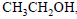the bond that undergoes heterolytic cleavage most readily is
Solution:
QUESTION: 32
Which of the following reaction intermediates contains three pairs of electrons in the valence shell of the reactive carbon?
Solution:
QUESTION: 33
Which of the following regents will not convert ethyl alcohol into ethyl chloride?
Solution:
QUESTION: 34
The reaction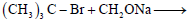produces mainly
Solution:
QUESTION: 35
1, 3-Dibromopropane reacts with metallic zinc to form
Solution:
QUESTION: 36
Molecular formula of Glauber’s salt is
Solution:
QUESTION: 37
Which alkene on ozonolysis gives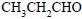and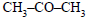?
Solution:
QUESTION: 38
Reaction of HBr with propene in the presence of peroxide gives
Solution:
QUESTION: 39
3-Phenylpropene on reaction with HBr gives (as major product)
Solution:
QUESTION: 40
Which of the following reactions will yield 2, 2-dibromopropane?
Solution:
QUESTION: 41
In the reaction below, X is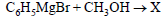Solution:
QUESTION: 42
Which salt on heating does not give brown coloured gas?
Solution:
QUESTION: 43
Plaster of Paris is
Solution:
QUESTION: 44
The right order of the solubility of sulphates of alkaline earth metals is
Solution:
QUESTION: 45
Which of the following gives propyne on hydrolysis?
Solution: